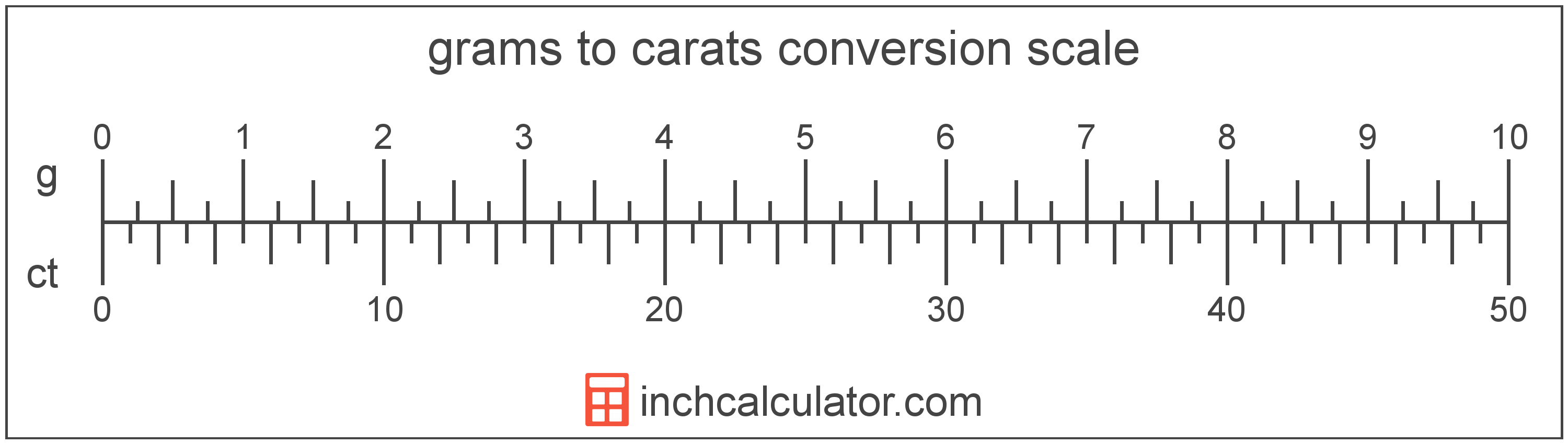Convert Grams to Carats

Enter the weight in grams below to get the value converted to carats.

Results in Carats:1 g = 5 ct
Do you want to convert carats to grams?

How to Convert Grams to CaratsTo convert a gram measurement to a carat measurement, multiply the weight by the conversion ratio. One gram is equal to 5 carats, so use this simple formula to convert:

carats = grams × 5

The weight in carats is equal to the grams multiplied by 5.

For example, here's how to convert 5 grams to carats using the formula above.
5 g = (5 × 5) = 25 ct

Grams and carats are both units used to measure weight. Keep reading to learn more about each unit of measure.

Grams

A gram is the mass/weight equal to 1/1,000 of a kilogram, and is roughly equal to the mass of one cubic centimeter of water.

The gram, or gramme, is an SI unit of weight in the metric system. Grams can be abbreviated as g, for example 1 gram can be written as 1 g.

Carats

The carat is a unit of mass/weight equal to 200 milligrams.

A carat is sometimes also referred to as a metric carat. Carats can be abbreviated as ct, and are also sometimes abbreviated as CD. For example, 1 carat can be written as 1 ct or 1 CD.

Gram Measurements and Equivalent Carat Conversions

Common gram values converted to the equivalent carat value
Grams Carats
1 g 5 ct
2 g 10 ct
3 g 15 ct
4 g 20 ct
5 g 25 ct
6 g 30 ct
7 g 35 ct
8 g 40 ct
9 g 45 ct
10 g 50 ct
11 g 55 ct
12 g 60 ct
13 g 65 ct
14 g 70 ct
15 g 75 ct
16 g 80 ct
17 g 85 ct
18 g 90 ct
19 g 95 ct
20 g 100 ct
21 g 105 ct
22 g 110 ct
23 g 115 ct
24 g 120 ct
25 g 125 ct
26 g 130 ct
27 g 135 ct
28 g 140 ct
29 g 145 ct
30 g 150 ct
31 g 155 ct
32 g 160 ct
33 g 165 ct
34 g 170 ct
35 g 175 ct
36 g 180 ct
37 g 185 ct
38 g 190 ct
39 g 195 ct
40 g 200 ct

More Gram Weight Conversions

US Customary & Imperial Units
Convert to Tons
1 g is equal to 1.1023E-6 tons
Convert to Pounds
1 g is equal to 0.002205 pounds
Convert to Ounces
1 g is equal to 0.035274 ounces
SI Units
Convert to Metric Tons
1 g is equal to 1.0E-6 metric tons
Convert to Kilograms
1 g is equal to 0.001 kilograms
Convert to Milligrams
1 g is equal to 1,000 milligrams
Other Units
Convert to Long Tons
1 g is equal to 9.8421E-7 long tons